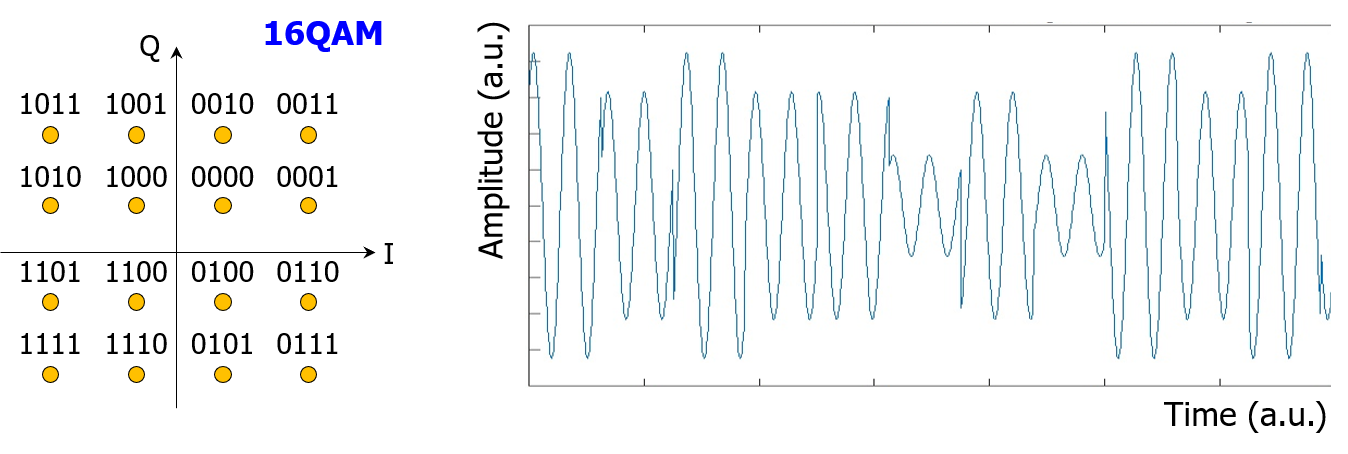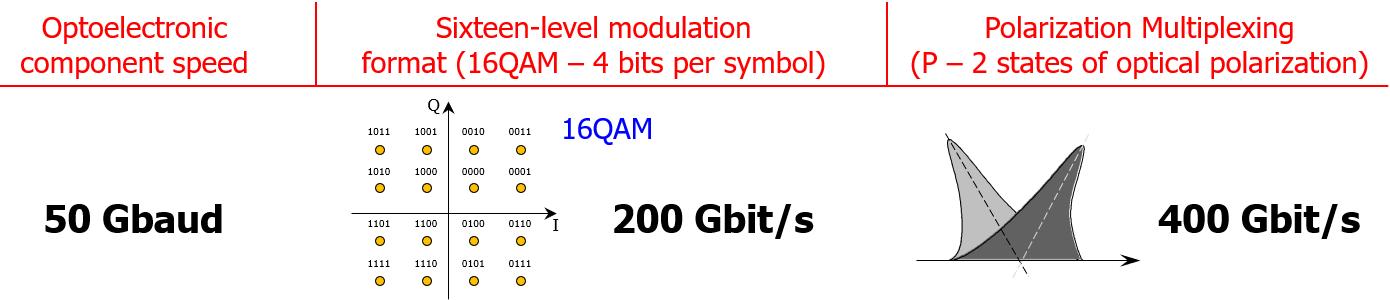# Single-Wavelength 400G Transmission Over 6,000 km

On 20 March 2018, ZTE, working in collaboration with OFS and NeoPhotonics, announced the transmission of single-wavelength 400G WDM channels over the impressive 6,000 km transmission length made of 100 km fiber spans, with 75 GHz channel spacing.  Which modulation format enabled this 400G data rate on a single wavelength?  What was the speed of the optoelectronic components inside the transponder to achieve this data rate?  And which factors drove the channel spacing of 75 GHz?

As always with multi-level modulation formats in coherent optical transmission, a little bit of math is required to understand what is behind the stage.  66 Gbaud optoelectronic components were used in this experiment.  This means that, e.g., the coherent driver modulator was capable to handle 66 giga symbols per second.

16QAM modulation format was also used.  In Quadrature Amplitude Modulation (QAM) format, the amplitudes of two waves delivered by the same optical source, 90° out-of-phase with each other (in quadrature), are modulated to represent the data signal.  Modulating the amplitude of two carriers in quadrature can be equivalently viewed as both modulating amplitude and phase of a single carrier (see figure below).  16QAM makes use of 16 distinct amplitude/phase symbols, which are usually represented under the format of a symbol constellation.  As four bits are required to encode 16 distinct symbols (0000 to 1111), each symbol of the 16QAM signal carries four bits.  The speed of 66 Gbaud (66 giga symbols per second) allows therefore to transmit 66 x 4 = 264 Gbit/s.The usual trick of optical polarization multiplexing was used: the polarized light emitted by the transmitter laser source was split on two optical paths; the optical wave on each path was modulated by the data stream and the lights from the two paths were recombined with orthogonal states of optical polarization.  In this way, the optical channel is made of two orthogonal states of optical polarization, each of them supporting a data stream.  This technique (Polarization Multiplexing or PM) doubles the number of bits per second carried by the PM-16QAM optical channel, bringing this number to 2 x 264 = 528 Gbit/s.  Given the need of overhead for powerful Forward Error Correction (FEC) code, a gross bit rate of 528 Gbit/s corresponds to a net bit rate of 400 Gbit/s.To summarize, we have the following steps for building this single-wavelength 400G optical channel:

• 66 Gbaud optoelectronic components -> 66 giga symbols per second
• 16QAM modulation format (4 bits/symbol) -> 66 x 4 = 264 Gbit/s
• Polarization multiplexing (PM-64QAM) -> 2 x 264 = 528 Gbit/s
• Removing the FEC overhead -> 400 Gbit/s

For simpler math and comparison between different modulation formats and symbol rate, net standard symbol rates are used, like in the figure below.ZTE press release is not very explicit but it looks like that probabilistic constellation shaping technique was used as well.  Probabilistic shaping imposes a non-uniform distribution on a set of equidistant constellation points; this technique enables to reduce the gap between the practically achieved capacity and the theoretically achievable Shannon’s capacity is reduced.  More details can be found in this post.

Also, it is very likely that Nyquist pulse-shaping was used to minimize the channel spacing, down to a figure close to the baud rate.  The spectral occupancy of the optical channel is driven by the symbol rate (here, 66 Gbaud).  With high-end digital signal processing and powerful Nyquist filtering, some recent experiments demonstrated channel spacing expressed in GHz only a few percent larger than the symbol rate expressed in GHz (e.g. channels spaced at 50 GHz, modulated at 49 Gbaud).  The channel spacing “performance” in ZTE’s demonstration looks suboptimal with 75 GHz channel spacing for a 66 Gbaud signal.

The attenuation characteristic of OFS TeraWave line fiber used in the 6,000 km demonstration was not provided in ZTE’s announcement but, betting on a figure of 0.158 dB/km, the 100 km span length lead to a 15.8 dB span loss, which exceeds the usual span loss of transoceanic cable systems.